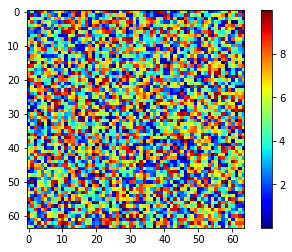# High training accuracy but false test when using training data

I’ve trained a simple CNN until the training accuracy is >99% (so it overfits but for now I’m testing m,y ability to push test images through a pretrained network).

However when I reuse an image from the training data to look at the output it’s the same classification for every image I try with the same ‘probabilities’ when using softmax.

The code looks as below (I’ve tried to simplify it to it’s key points to see if I’m missing something really obvious).

``````tester = torch.load(IMG_PATH+'CategoricalNet.pt')

print(tester)

``````

CategoricalNet(
(feature_extractor): Sequential(
(0): Conv2d(1, 64, kernel_size=(5, 5), stride=(1, 1), padding=(2, 2))
(1): ReLU()
(2): Conv2d(64, 128, kernel_size=(5, 5), stride=(1, 1), padding=(2, 2))
(3): ReLU()
(4): BatchNorm2d(128, eps=1e-05, momentum=0.1, affine=True, track_running_stats=True)
(5): MaxPool2d(kernel_size=2, stride=2, padding=0, dilation=1, ceil_mode=False)
(6): Conv2d(128, 256, kernel_size=(5, 5), stride=(1, 1), padding=(2, 2))
(7): ReLU()
(8): BatchNorm2d(256, eps=1e-05, momentum=0.1, affine=True, track_running_stats=True)
(9): MaxPool2d(kernel_size=2, stride=2, padding=0, dilation=1, ceil_mode=False)
)
(classifier): Sequential(
(0): Dropout(p=0.25)
(1): Linear(in_features=65536, out_features=256, bias=True)
(2): ReLU()
(3): Dropout(p=0.25)
(4): Linear(in_features=256, out_features=10, bias=True)
)
)

``````test = fits.open("E:/Documents/Python_Scripts/CNN/TRAINING/EXAMPLE_DATA.fits")
transform = transforms.Compose([transforms.ToTensor(), transforms.Normalize(, )])
data = transform(d.reshape(*d.shape, 1)).unsqueeze(0).float().cuda()

output = torch.nn.functional.softmax(tester(data),dim=1).cpu().detach().numpy()

print(output)
``````
``````TRUE LABEL= 5

[[0.10622309 0.1435124  0.05875074 0.0495275  0.06827779 0.03227602
0.17474921 0.17845923 0.15276037 0.03546367]]

TEST LABEL=  7
``````

And similarly for another test case:

``````TRUE LABEL= 0

[[0.10622309 0.1435124  0.05875074 0.0495275  0.06827779 0.03227602
0.17474921 0.17845923 0.15276037 0.03546367]]

TEST LABEL= 7
``````

I’ve checked that the image transformation matches that in the training:

``````transform = transforms.Compose([transforms.ToTensor(), transforms.Normalize(, )])
``````

So I’m not sure why the predictions would be the same for every test case, any help on this matter would be greately appreciated!

Try tester = tester.eval()
Otherwise the learned batch norm parameters aren’t used

Unfortunately I gave that a try and it doesn’t seem to change the problem. I use .eval() before saving the network (which is done using:

`torch.save(model, IMG_PATH+'CategoricalNet.pt')`

and then repeat .eval() when loading in the network again just to be sure.

Thanks for the reply by the way!

So if it’s the same data and the same network, there must be some kind of discrepancy in your training vs test code.

I suggest the following check: first remove shuffle so you can load the train and “test” (which is just the train folder) in the same order. Print out torch.norm(data) for both cases, (actually verify they are the same input to the network). If they are, and the output is different, try to step through the layer weights to see which are different. Good luck!

1 Like

Thanks for the suggestions, I’ll give them a try now and see what I can findIt looks like I was mishandling the transformations, as outputting the normalised test data doesn’t seem to have scaled the values:

``````data = np.random.uniform(0,10,[64,64])
transform = transforms.Compose([transforms.ToTensor(), transforms.Normalize(,)])
d = transform(data.reshape(*data.shape, 1)).unsqueeze(0).float().cuda()
output = torch.nn.functional.softmax(tester(d),dim=1).cpu().detach().numpy()
plt.figure()
plt.imshow(d[0,0,:,:],cmap='jet')
plt.colorbar()
``````Upon doing more research, I’ve figured out that this is because the scaling does the operation: (x-0)/1 which of course makes no change to the data